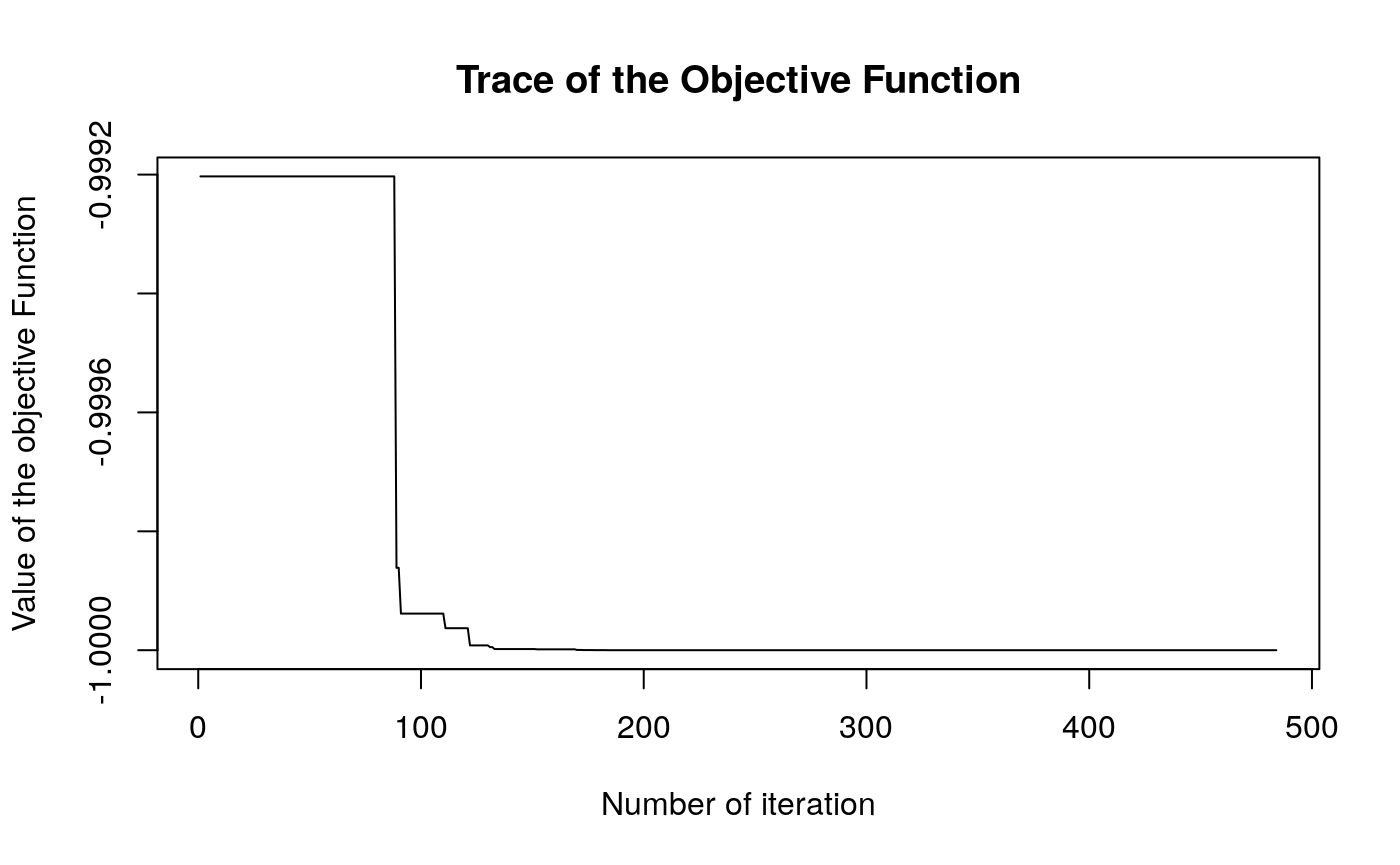This is an implementation of Karaboga (2005) ABC optimization algorithm. It was developed upon the basic version programmed in C and distributed at the algorithm’s official website (see the references).

Any evident (precision) error should be blamed to the package author (not to the algorithm itself).

# Example

library(ABCoptim)

# Function to optimize. Min at (pi,pi)
fun <- function(x) {
-cos(x)*cos(x)*exp(-((x - pi)^2 + (x - pi)^2))
}

# Since it is stochastic, we need to set a seed to get the same
# results.
set.seed(123)

# Finding the minimum
ans <- abc_optim(rep(10,2), fun, lb=-20, ub=20, criter=200)
ans
##
##  An object of class -abc_answer- (Artificial Bee Colony Optim.):
##  par:
##     x:  3.141593
##     x:  3.141593
##
##  value:
##           -1.000000
##
##  counts:
##            484
plot(ans)